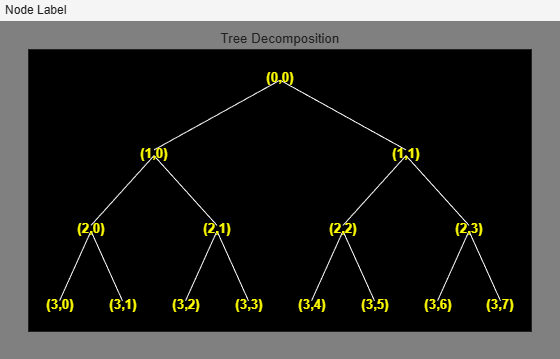# ind2depo

Node index to node depth-position

## Syntax

```[D,P] = ind2depo(ORD,[D P]) ```

## Description

`ind2depo` is a tree-management utility.

For a tree of order `ORD`, ```[D,P] = ind2depo(ORD,N)``` computes the depths `D` and the positions `P` (at these depths `D`) for the nodes with indices `N`.

The nodes are numbered from left to right and from top to bottom. The root index is 0.

`N` must be a column vector of integers ```(N ≥ 0)```.

Note that `[D,P] = ind2depo(ORD,[D P])`.

## Examples

collapse all

Create a binary wavelet packet tree with three levels.

```Ord = 2; Lev = 3; T = ntree(Ord,Lev);```

Plot the binary wavelet packet tree.

`plot(T)`Obtain the indices of the nodes in linear order.

`idx = allnodes(T);`

Convert the indices to depth-position format.

```[depth,pos] = ind2depo(Ord,idx); table(depth,pos)```
```ans=15×2 table depth pos _____ ___ 0 0 1 0 1 1 2 0 2 1 2 2 2 3 3 0 3 1 3 2 3 3 3 4 3 5 3 6 3 7 ```

## Version History

Introduced before R2006a